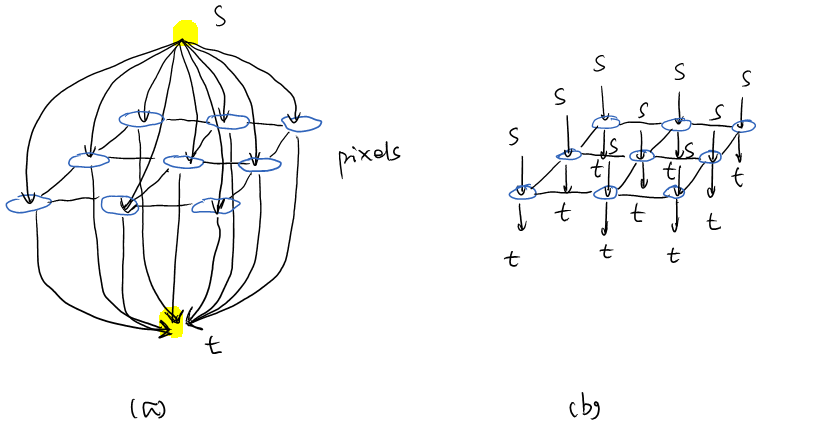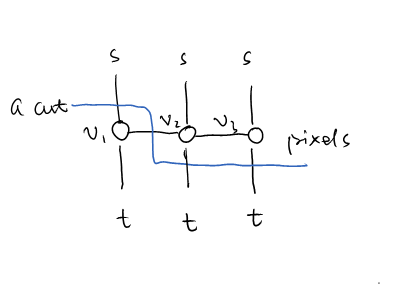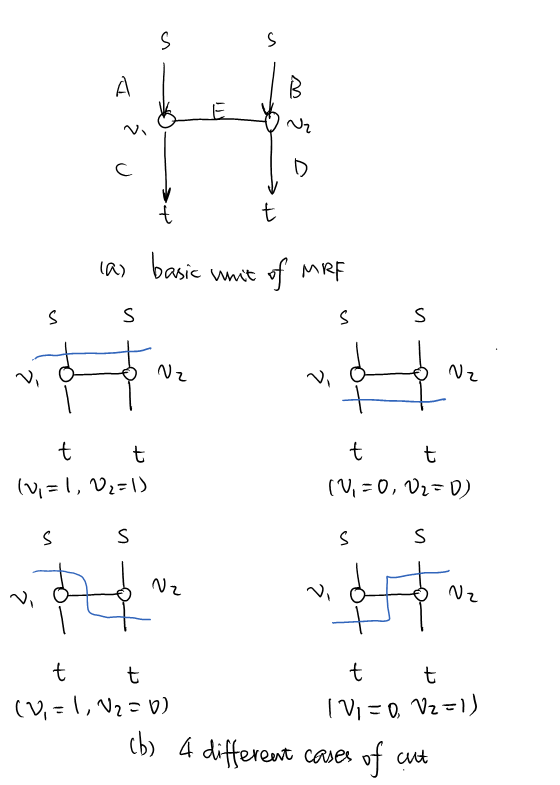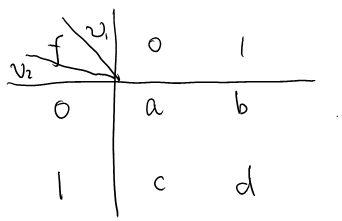## Markov Random Field (MRF) and Graph-Cut (2)

In the previous post, we've seen applications of MRF in image restoration. This post will illustrate some more insights on MRF and graph-cut, a fast solution for global optimal.

## MRF, graph, and graph cut

In the last blog post, we built a graph whose cut equals our energy function plus a constant. Therefore we can get the global optimal of our energy function by solving the graph's min-cut. But is this approach extendable? Can we minimize any energy functions via graph-cut? Even if it's not possible to optimize any functions, it'll still be great if we can find a general way to construct such a graph to optimize some certain energy functions on MRF.

Follow the motivation from last post, a graph can be constructed from MRF intuitively. To formulate it as a flow-cut problem, there need two sets of vertices. First is the vertices for pixels, each vertex corresponding to one pixel, and adjacent pixels connected with an undirected weighted edge. Second is a set of two auxiliary vertices, the source s and sink t. The graph looks like Fig 1 (a). And actually if we mainly focus on the middle layer, i.e. the pixel vertices layer, it looks like Fig 1 (b).Fig. 1. graph construction for MRF. (a) shows all the vertices and edges, while (b) shows only the pixel vertices.

To make it clear, we further restrict to observe one row of pixel vertices, as Fig. 2 shows. Then a cut means every vertex needs to make a choice, following s or t. That is, if we call the edges to s or v as t-links, and edges between two pixel vertices as n-links, for every pixel vertex v, a cut must cross one of its two t-links. But for n-links, a cut will only cross the boundaries of different choices, like ($v_2, v_3$).Fig 2. one row of pixels in the graph with a cut (in blue).

So that we can see, the problem of finding a min-cut in such a graph, is actually forcing every pixel to make a choice from two labels (s or t), to minimize 1) the cost of choosing labels, plus 2) penalty of adjacent choice discontinuity. Obviously the combinational solution space is O(2^n), but fortunately we have polynomial time (and actually very fast) algorithms to get global optimal. (Why could we do so? I guess that's because the solution space has very special property, but cannot tell what it is exactly. Any ideas?)

## What functions can be minimized via graph-cut

If we look even closer, it's easy to find the basic unit of MRF is actually a pair of vertices (i.e. a clique. All the cliques in the pixel vertices level only contain two nodes). As Fig. 3 shows, if we try to cut this unit, there are only 4 possible approaches.Fig 3. Basic unit of MRF and 4 cases of a cut. The capital letters in (a) indicate the weights.

Therefore, to minimize a certain energy function, what we need to do is just determine the weights A, B, C, D and E (in every pair), to make the energy function equal to cut value on every possible combination of $(v_1, v_2)$. Considering the energy function is given, it's actually fairy easy.

Table 1. Values of energy functionFor clarity, let's give the energy function values some alias as Table 1 shows. To make the cut values equal to function values, we have

$$\begin{cases}A + B = d & (1) \\ C + D = a & (2) \\ A + E + D = b & (3) \\ B + E + C = c & (4)\end{cases}$$

Given the capability of edges a graph must be non-negative, we can easily get $b + c \geq a + d$ by (3) + (4) - (1) - (2). Therefore this is a necessary condition to minimize a function $f$ via graph cut. In addition, if $b + c \geq a + d$ satisfies, it can be proved that we can always construct a solution satisfying $A, B, C, D, E \geq 0$. For example, when $a \geq |b - c|$, we can set

$$\begin{cases}A = d / 2\\ B = d / 2\\ C = (a - b + c) / 2\\ D = (a + b - c) / 2\end{cases}$$

Otherwise we can always adjust some preconditions (such as $A = B$ in this solution) to find other feasible solutions.

So in summary, an energy function can be minimized if and only if $f(0, 0) + f(1, 1) \leq f(0, 1) + f(1, 0)$. And when given a certain function, we can use the equations (1) to (4) to find proper weights to minimize it with graph-cut. (For more strict proof please consult )

## More applications

MRF is naturally suitable for foreground/background segmentation. By using Gaussian metrics $E(v_1, v_2) = \exp(||v_1 - v_2|| / 2\sigma ^2)$, MRF performs well in segmentation tasks. Introducing user input is also simple. To enable users specify which area to be foreground/background, we only need to make the capacity of one out of two t-links very large for such "priori" vertices, so that they will only be labeled as we wish.

A demo video can be found below. In this video, we first specify some area as background with green strokes, and then some other regions as foreground with red strokes. We can see how MRF achieve good performance in segmentation.

## References

 V. Kolmogorov and R. Zabih, "What energy functions can be minimized via graph cuts?," IEEE transactions on pattern analysis and machine intelligence, vol. 26, Feb. 2004, pp. 147-59.

 Y. Boykov and G. Funka-Lea, “Graph Cuts and Efficient N-D Image Segmentation,” International Journal of Computer Vision, vol. 70, Nov. 2006, pp. 109-131.